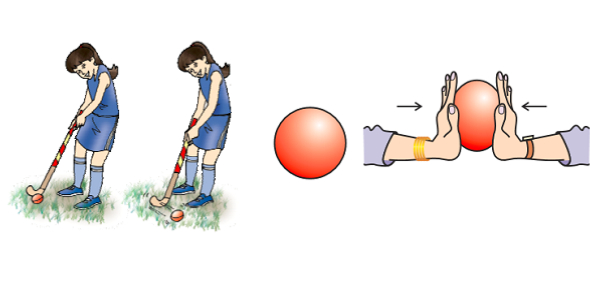# Force And Motion Quiz Questions: Trivia!

11 Questions | Total Attempts: 260SettingsForce and motion pertain to one another. You cannot have motion without force. If you create an applied force, it makes objects move. For this quiz, you shall be responsible for understanding how force is measured, what the law of universal gravitation says, what Newton’s first, second, and third law describes, and what refers to an object’s velocity. This quiz will make you knowledgeable in the subject of force and motion. Go for it.

• 1.
Force is a push or a pull, measured in:
• A.

Joules.

• B.

Watts.

• C.

Newtons.

• D.

Kilograms.

• E.

Meters.

• 2.
Gravity is an attractive force, and depends on _______ and __________
• A.

Weight, distance.

• B.

Mass, distance.

• C.

Force, mass.

• D.

Distance, time.

• 3.
The Law of Universal Gravitation says
• A.

Objects with mass exert gravitational pull.

• B.

The greater an object's mass, the greater its gravity.

• C.

The closer two objects are, the greater their gravitational attraction.

• D.

All of the above.

• 4.
In order to determine the force, what measurements must you make?
• A.

Weight and height.

• B.

Weight and distance.

• C.

Distance and acceleration.

• D.

Mass and acceleration.

• 5.
If the mass of an object remains constant, and you increase the force applied to it, what happens to the object's acceleration?
• A.

Acceleration remains constant.

• B.

Acceleration increases.

• C.

Acceleration decreases.

• D.

There's not enough information to decide.

• 6.
Newton's 1st Law describes:
• A.

The relationship between mass, acceleration, and force.

• B.

Balanced and unbalanced forces.

• C.

The tendency of objects to resist changes in motion.

• D.

Equal and opposite forces.

• 7.
Newton's 2nd Law describes:
• A.

The relationship between mass, acceleration, and force.

• B.

Balanced and unbalanced forces.

• C.

The tendency of objects to resist motion.

• D.

Equal and opposite forces.

• 8.
Newton's 3rd Law describes:
• A.

The relationships between mass, acceleration, and force.

• B.

Balanced and unbalanced forces.

• C.

The tendency of objects to resist changes in motion

• D.

Equal and opposite forces.

• 9.
Once an object is in motion, we can determine its average speed by measuring:
• A.

The distance traveled and how long it took to get there.

• B.

The difference between its final speed and the initial speed.

• C.

The distance traveled and its rate of acceleration.

• D.

The direction it traveled and how long it took to get there.

• 10.
Which of the following refers to an object's velocity?
• A.

Increasing distance from a reference point over time.

• B.

Decreasing distance from a reference point over time.

• C.

Constantly changing the direction of travel over time.

• D.

All of the above.

• 11.
You can describe an object as accelerating when:
• A.

The object increases its velocity.

• B.

The object decreases its velocity.

• C.

The object changes direction.

• D.

All of the above.

Related TopicsBack to top